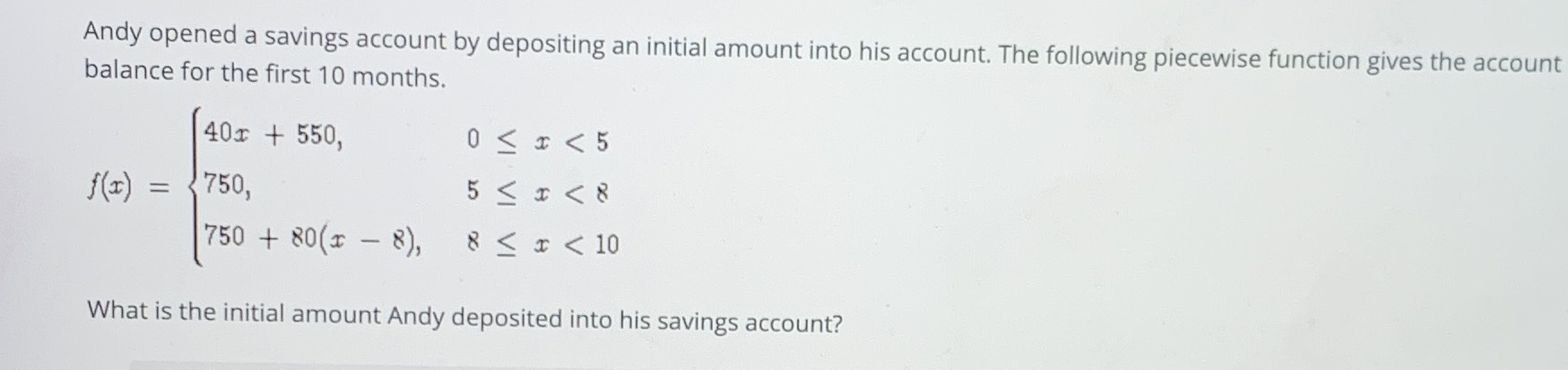### Still have math questions?

Algebra
QuestionAndy opened a savings account by depositing an initial amount into his account. The following piecewise function gives the account balance for the first $$10$$ months.

$$f ( x ) = \left \{ \begin{array} { l l } { 40 x + 550 , } & { 0 \leq x < 5 } \\ { 750 , } & { 5 \leq x < 8 } \\ { 750 + 80 ( x - 8 ) , } & { 8 \leq x < 10 } \end{array} \right .$$

What is the initial amount Andy deposited into his savings account?